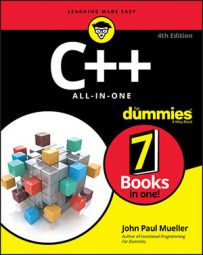##### C++ All-in-One For DummiesRandom number generators fulfill a number of purposes. Everything from games to simulations require a random number generator to work properly. Randomness finds its way into business what-if scenarios as well. In short, you need to add random output to your application in many situations.

Creating a random number isn’t hard. All you need to do is call a random number function as shown in the RandomNumberGenerator example:

```#include <iostream>
#include <time.h>
#include <stdlib.h>
using namespace std;
int main()
{
// Always set a seed value.
srand((unsigned int)time(NULL));
int RandomValue = rand() % 12;
cout << "The random month number is: " << RandomValue + 1 << endl;
return 0;
}```

Actually, not one of the random number generators in the Standard Library works properly — imagine that! They are all pseudorandom number generators: The numbers are distributed such that it appears that you see a random sequence, but given enough time and patience, eventually the sequence repeats.

In fact, if you don’t set a seed value for your random number generator, you can obtain predictable sequences of numbers every time. How boring. Here is typical output from this example:

`The random month number is: 7`

The first line of code in main() sets the seed by using the system time. Using the system time ensures a certain level of randomness in the starting value — and therefore a level of randomness for your application as a whole. If you comment out this line of code, you see the same output every time you run the application.

The example application uses rand() to create the random value. When you take the modulus of the random number, you obtain an output that is within a specific range — 12 in this case. The example ends by adding 1 to the random number because there isn’t any month 0 in the calendar, and then outputs the month number for you.

The Standard Library provides access to two types of pseudorandom number generators. The first type requires that you set a seed value. The second type requires that you provide an input value with each call and doesn’t require a seed value. Each generator outputs a different data type, so you can choose the kind of random number you obtain.

The table lists the random number generators and tells you what data type they output.

Pseudorandom Number Generator Functions
Function Output Type Seed Required?
rand integer yes
drand48 double yes
erand48 double no
lrand48 long yes
nrand48 long no
mrand48 signed long yes
jrand48 signed long no

Now that you know about the pseudorandom number generators, look at the seed functions used to prime them. The following table lists the seed functions and their associated pseudorandom number generator functions.

Seed Functions
Function Associated Pseudorandom Number Generator Function
srand rand
srand48 drand48
seed48 mrand48
lcong48 lrand48